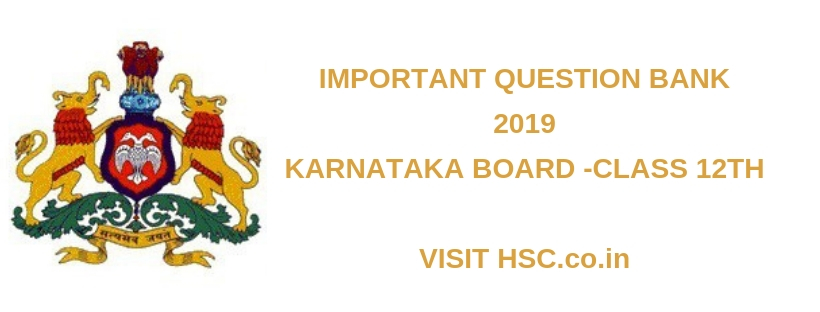# Physics – Important Question Bank for Karnataka Intermediate II PUC (HSC) Board Exam 2019

HSC Board Exams are fast approaching and students are getting anxious about how to prepare for their HSC Board Exams. So we had mentioned some HSC Study Tips to help students in Cracking HSC Exams.

After the tremendous success of our last year Important Question Bank for Karnataka 12th std  PUC HSC Board Exam 2016 2017 and 2018 we have also created a list of Important Question Bank for Karnataka Intermediate II PUC (HSC) Board Exam 2019 which are likely to appear in HSC Board Exams this year.To unlock the content Click on any of 3 buttons available in the box below saying “This content is Locked”. Once you click on the button the content will get unlocked on same page itself. You must click on social media button showing in below box ie Facebook, Twitter or Google Plus to unlock the content.

1. If this combination is connected to a battery of emf 10V and negligible internal resistance, obtain the potential drop across each resistor.
2. Define and explain Gauss law in magnetism.
3. A convex lens of focal length 0.24m and of refractive index 1.5 is completely immersed in water of refractive index 1.33. Find the change in the focal length of the lens.
4. Three resistors of resistances 2ohms, 3ohms and 4ohms are combined in series. What is the total resistance of the combination?
5. Arrive at the expression for velocity selector using Lorentz force.
6. When two capacitors are connected in series and connected across 4kV line, the energy stored in the system is 8J. The same capacitors, if connected in parallel across the same line, the energy stored are 36J. Find the individual capacitances.
7. Derive the relation between electric field and electric potential.
8. Derive an expression for equivalent focal length of two thin lenses kept in contact.
9. Derive an expression for equivalent emf and equivalent internal resistance when two cells are connected in parallel.
10. What is rectification? With relevant circuit diagram and waveforms explain the working of p-n junction diode as a full-wave rectifier.
11. What is interference of light? Arrive at the conditions for constructive and destructive interference by assuming the expression for intensity.
12. Write the experimental observations of photoelectric effect.
13. What is photoelectric effect? Using Einstein’s photoelectric equation, explain three experimental results.
14. Derive an expression for electric field due to an electric dipole at a point on the axial line.
15. What is meant by self inductance and mutual inductance?
16. Using Huygen’s wave theory of light, derive Snell’s law of refraction
17. Derive an expression for the force between two parallel conductors carrying currents. Also define ampere.
18. Write the relation between the path difference and wavelength of light wave used for constructive and destructive interference of light.
19. Obtain the expression for the magnetic energy stored in a coil
20. Deduce the condition for balance of a Wheatstone’s bridge using Kirchhoff’s rules.
21. Monochromatic light of wavelength 5000 A° from a narrow slit is incident on the double slit. If the separation of 10 fringes on the screen 1 m away is 2 cms. Find the slit separation
22. Write the properties of ferromagnetic materials.
23. Deduce the expression for total energy of electron in the nth stationary orbit of hydrogen atom
24. Derive an expression for drift velocity of free electrons in a conductor.
25. What are matter waves? Write the expression for de-Brogile wavelength of a particle and explain the terms.
26. What is an amplifier? Draw the simple circuit of transistor amplifier in CE mode.
27. With a diagram, explain the working of a transformer.
28. Distinguish between paramagnetic and ferromagnetic substances.

Hi, we’re trying to collate and gather the data and would be updating it here a few days before the exam. Please keep on visiting our website for updates.

Please use the comments box below and post questions that you think are important from your analysis. It would help the HSC community a lot.

Do subscribe to our updates so that you do not miss out on any important information that we push your way.

Don’t forget to read : MUST REMEMBER THINGS on the day of Exam for HSC Students